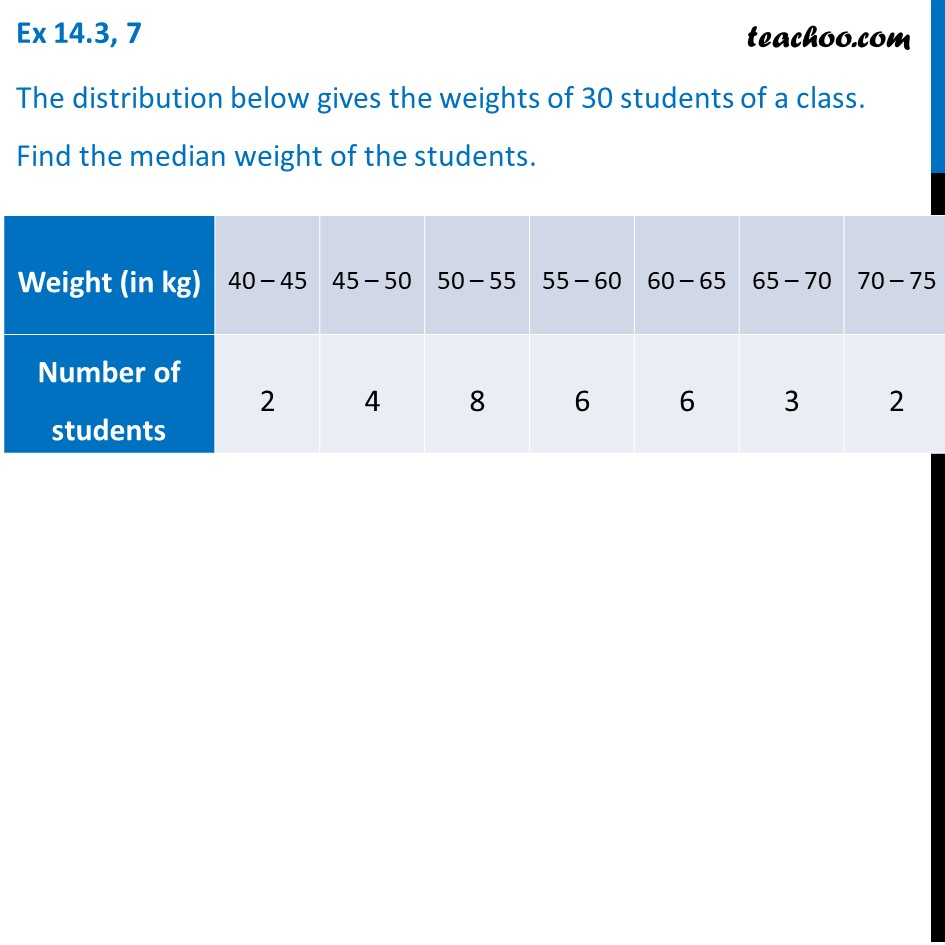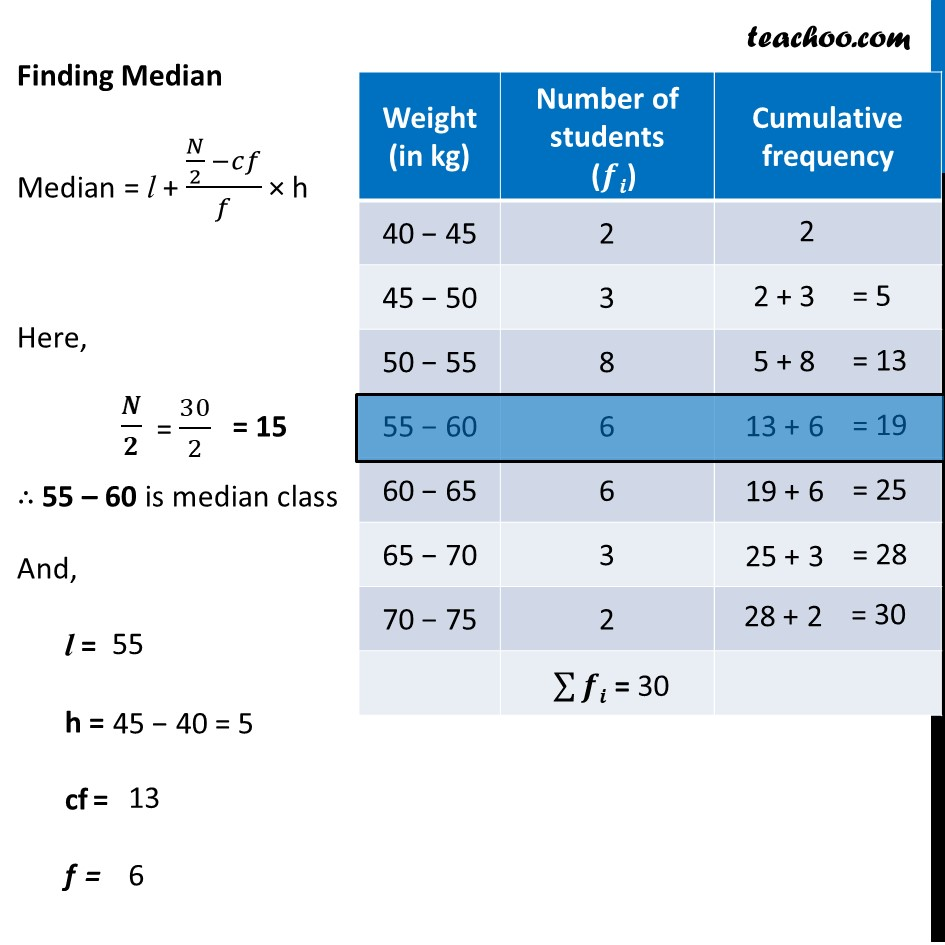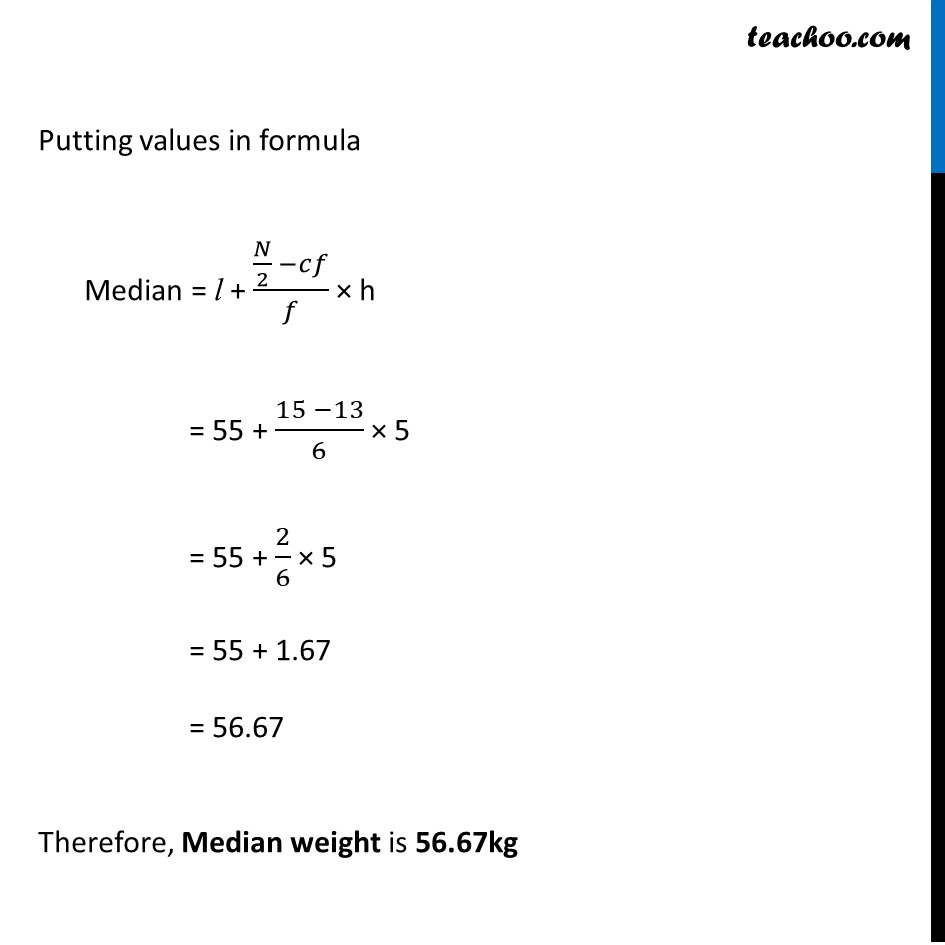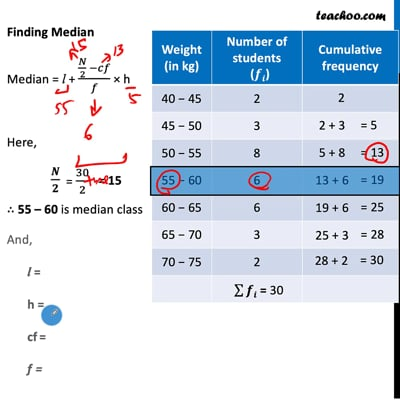Ex 14.3

Chapter 14 Class 10 Statistics (Term 2)
Serial order wiseThis video is only available for Teachoo black users

### Transcript

Ex 14.3, 7 The distribution below gives the weights of 30 students of a class. Find the median weight of the students. Finding Median Median = l + (𝑁/2 −𝑐𝑓)/𝑓 × h Here, 𝑵/𝟐 ∴ 55 – 60 is median class And, l = h = cf = f = Putting values in formula Median = l + (𝑁/2 −𝑐𝑓)/𝑓 × h = 55 + (15 −13)/6 × 5 = 55 + 2/6 × 5 = 55 + 1.67 = 56.67 Therefore, Median weight is 56.67kg